# Boolean Algebra Theorems and Laws of Boolean Algebra

## What is Boolean Algebra?

Boolean algebra is a different kind of algebra or rather can be said a new kind of algebra which was invented by world-famous mathematician George Boole in the year of 1854. He published it in his book “An Investigation of the Laws of Thought”.

Later using this technique Claude Shannon introduced a new type of algebra which is termed as Switching Algebra. In digital electronics, there are several methods of simplifying the design of logic circuits. This algebra is one of these methods.

According to George Boole symbols can be used to represent the structure of logical thoughts. This type of algebra deals with the rules or laws, which are known as laws of Boolean algebra by which the logical operations are carried out.

There are also few theorems of Boolean algebra, that are needed to be noticed carefully because these make calculation fastest and easier. Boolean logic deals with only two variables, 1 and 0 by which all the mathematical operations are to be performed.

Boolean algebra or switching algebra is a system of mathematical logic to perform different mathematical operations in a binary system. There only three basis binary operations, AND, OR, and NOT by which all simple, as well as complex binary mathematical operations, are to be done.

There are many rules in Boolean algebra by which those mathematical operations are done. In Boolean algebra, the variables are represented by English Capital Letter like A, B, C, etc and the value of each variable can be either 1 or 0, nothing else.

In Boolean algebra, an expression given can also be converted into a logic diagram using different logic gates like AND gate, OR gate and NOT gate, NOR gates, NAND gates, XOR gates, XNOR gates, etc.

Some basic logical Boolean operations,
AND OperationOR Operation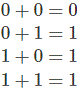Not Operation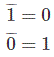Some basic laws for Boolean AlgebraA . 0 = 0 where A can be either 0 or 1.
A . 1 = A where A can be either 0 or 1.
A . A = A where A can be either 0 or 1.
A . Ā = 0 where A can be either 0 or 1.
A + 0 = A where A can be either 0 or 1.
A + 1 = 1 where A can be either 0 or 1.
A + Ā = 1
A + A = A
A + B = B + A where A and B can be either 0 or 1.
A . B = B . A where A and B can be either 0 or 1.
The laws of Boolean algebra are also true for more than two variables like,

### Cumulative Law for Boolean Algebra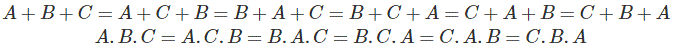According to Cumulative Law, the order of OR operations and AND operations conducted on the variables makes no differences.

### Associative Laws for Boolean Algebra

This law is for several variables, where the OR operation of the variables result is the same through the grouping of the variables. This law is quite the same in the case of AND operators.### Distributive Laws for Boolean Algebra

This law is composed of two operators, AND and OR.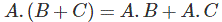Let us show one use of this law to prove the expression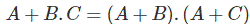Proof: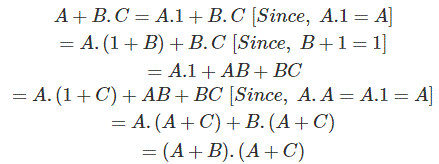### Redundant Literal RuleFrom the truth table,

 Inputs Output A B ĀB A + ĀB 0 0 0 0 0 1 1 1 1 0 0 1 1 1 0 1
 Inputs Output A B A+B 0 0 0 0 1 1 1 0 1 1 1 1

From the truth table, it is proved that,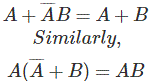### Absorption Laws for Boolean Algebra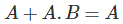Proof from truth table,

 Inputs Output A B AB A+A.B 0 0 0 0 0 1 0 0 1 0 0 1 1 1 1 1

Both A and A+A.B column is the same.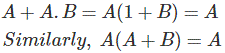Proof from truth table,

 A B A+B A.X(A+B) 0 0 0 0 0 1 1 0 1 0 1 1 1 1 1 1

Both A and A.X or A(A+B) columns are the same.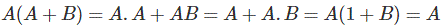From De Morgan’s Theorem,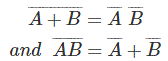Proof from truth table,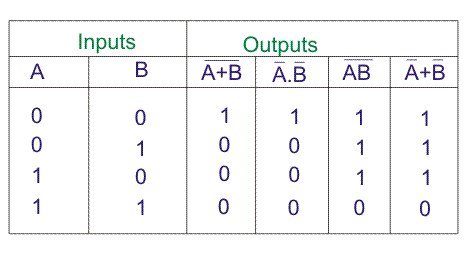## Examples of Boolean Algebra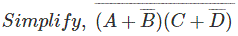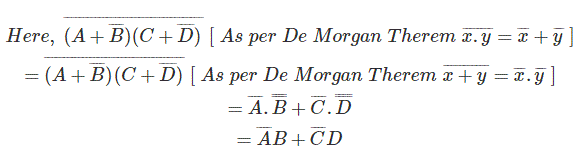These are another method of simplifying a complex Boolean expression. In this method, we only use three simple steps.

1. Complement entire Boolean expression.
2. Change all ORs to ANDs and all ANDs to ORs.
3. Now, complement each of the variables and get the final expression.

By this method,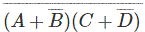will be first complemented, i.e.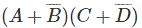.Now, change all (+) to (.) and (.) to (+) i.e.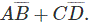Now, complement each of the variables,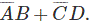This is the final simplified form of a Boolean expression,And it is exactly equal to the results which have been come by applying De Morgan Theorem.
Another example,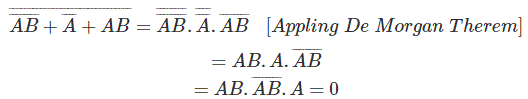By the Second Method,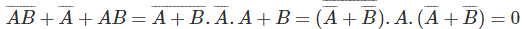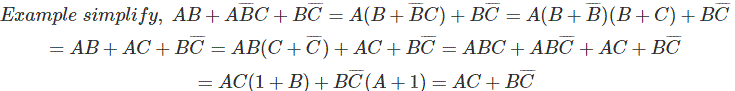Representation of Boolean function in the truth table.
Let us consider a Boolean function,Now let us represent the function in the truth table.Thus we have shown some basic laws of Boolean algebra. On the other page, we have described De Morgan’s theorems and related laws on it.

Want To Learn Faster? 🎓
Get electrical articles delivered to your inbox every week.
No credit card required—it’s 100% free.